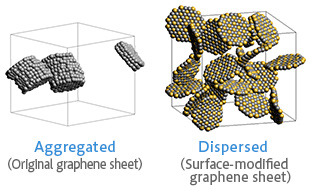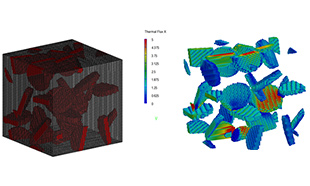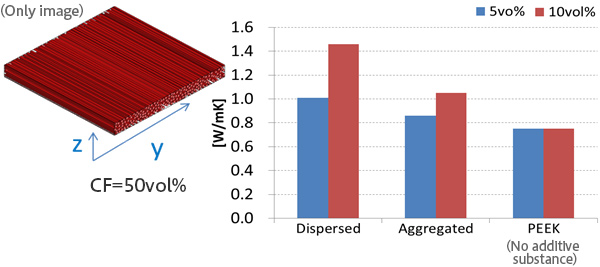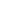DPD / Mean Field
Continuum Model
Multiscale Analysis
Thermal
Interface / Phase Separation / Particle Dispersion
Materials Science

# Thermal analysis which considers the dispersibility of graphene sheets

Modification of thermal conductivity of CFRTP matrix by adding graphene sheets
by using J-OCTA and Digimat.

## Purpose and Method

By adding graphene sheets, the thermal conductivity of CFPRP material can be improved. However, when unmodified graphene sheets are added, they aggregate and then the thermal conductivity may not be improved as much as expected. In this example, the objective of the simulation is for understanding the effect of the dispersed structure of graphene sheets to materials. The matrix is assumed to be PEEK.

First, compute how much the surface modification of graphene sheets changes the dispersibility, by using J-OCTA. (See Figure 1)

Next, use the coordinates of the graphene sheet computed by J-OCTA as the input for Digimat-FE/Modeler to recreate the microstructure model. Then, calculate the thermal conductivity by using Digimat-FE/Solver. (See Figure 2)

Finally, by using the thermal conductivity which Digimat-FE/Solver calculated, compute the averaged thermal conductivity of the whole CFRTP with considering the effect from the dispersed graphene sheet. (Digimat-MF, see Figure 3)Figure 1: Dispersed structure - J-OCTA (Graphene 10vol%)Figure 2: FEM model - Digimat-FE/Modeler (left), Thermal analysis result - Digimat-FE/Solver (right)
(Heat flux contour)

## Simulation result

The simulation demonstrated that the difference of the filler dispersed structure in the matrix influences the thermal conductivity of the whole material.

The thermal conductivity of CFRTP was successively improved by adding graphene sheets to the matrix. Furthermore, we can see that the graphene sheet whose surface is modified and dispersed uniformly can improve the thermal conductivity of CFRTP more effectively than the one unevenly dispersed, regardless of the amount of the added substances.Figure 3 : Thermal analysis result for the whole CFRTP using Digimat-MF. (averaged in y-, x-axis)

• Source
• T.Ozawa, T.Nakayama, M.Watanabe, G.Goldbeck, V.Regnier, MOLMOD2015This website uses cookies to improve functionality and performance. If you continue browsing the site, you are giving implied consent to the use of cookies on this website. If you want to know more or refuse consent, read our Cookie Policy.

Accept